# Tangent space

The tangent space of a differentiable manifold M is a vector space at a point p on the manifold whose elements are the tangent vectors (or velocities) to the curves passing through that point p. The tangent space at this point p is usually denoted TpM.

The tangent space is necessary for a manifold because it offers a way in which tangent vectors at different points on the manifold can be compared (via an affine connection). If the manifold is a hypersurface of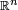$\mathbb{R}^n$, then the tangent space at a point can be thought of as a hyperplane at that point. However, this ambient Euclidean space is unnecessary to the definition of the tangent space.

The tangent space at a point has the same dimension as the manifold, and the union of all the tangent spaces of a manifold is called the tangent bundle, which itself is a manifold of twice the dimension of M.

## Definition

Although it is tempting to define a tangent space as a "space where tangent vectors live", without a definition of a tangent space there is no definition of a tangent vector. There are various ways in which a tangent space can be defined, the most intuitive of which is in terms of directional derivatives; the space TpM is the space identified with directional derivatives at p.

### Directional derivative

A curve on the manifold is defined as a differentiable map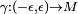$\gamma: (-\epsilon,\epsilon) \rightarrow M$. Let$\gamma(0) \, = \, p$. If one defines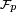$\mathcal{F}_p$ to be all the functions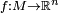$f:M \rightarrow \mathbb{R}^n$ that are differentiable at the point p, then one can interpret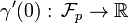$\gamma'(0): \, \mathcal{F}_p \rightarrow \mathbb{R}$

to be a linear functional such that$\gamma'(0)(f) = (f \circ \gamma)'(0) = \lim_{h \rightarrow 0} \frac{f(\gamma(h)) - f(\gamma(0))}{h}$

and is a directional derivative of f in the direction of the curve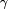$\gamma$. This operator can be interpreted as a tangent vector. The tangent space is then the set of all directional derivatives of curves at the point p.

### Directional derivatives as a vector space

If this definition is reasonable, then the directional derivatives, must form a vector space of the same dimension as the n-dimensional manifold M. The easiest way to show this is to show that some n directional derivatives form a basis of the vector space, and in order to do so, one needs to introduce a coordinate chart (see differentiable manifold).

Let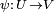$\psi: \, U \, \rightarrow V$ where$U \subset M$,$V \subset \mathbb{R}^n$ be a coordinate chart, and$\psi \,=\, (x_1, \cdots,\, x_n)$. The most obvious candidates for basis vectors would be the directional derivatives along the coordinate curves, i.e. the i-th coordinate curve would be$\gamma_i = \psi^{-1} (\psi(p) + te_i) \$

where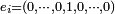$e_i = (0, \cdots, 0 , 1 , 0, \cdots, 0)$, the 1 being in the i-th position.

The directional derivative along a coordinate curve can be represented as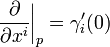$\frac{\partial}{\partial x^i}\bigg|_{p} = \gamma_i '(0)$

because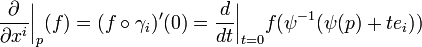$\frac{\partial}{\partial x^i}\bigg|_{p} (f) = (f\circ \gamma_i)'(0) = \frac{d}{dt} \bigg|_{t=0} f(\psi^{-1} (\psi(p) + te_i))$

which becomes, via the chain rule,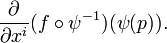$\frac{\partial}{\partial x^i} (f \circ \psi^{-1})(\psi(p)).$

For an arbitrary curve$\gamma, \, \psi \circ \gamma \, = \, (\gamma_1, \, \cdots, \, \gamma_n),$ then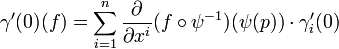$\gamma'(0)(f) = \sum_{i=1}^{n} \frac{\partial}{\partial x^i} (f \circ \psi^{-1})(\psi(p)) \cdot \gamma_i'(0)$

which is simply$\sum^{n}_{i=1} \gamma'_{i}(0) \cdot \frac{\partial}{\partial x^i}\bigg|_{p} (f)$

so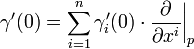$\gamma'(0) = \sum^{n}_{i=1} \gamma'_{i}(0) \cdot \frac{\partial}{\partial x^i}\bigg|_{p}$

as f is arbitrary.Some content on this page may previously have appeared on Citizendium.